### Home > CALC > Chapter 12 > Lesson 12.2.4 > Problem12-100

12-100.
1. Use Taylor polynomials to quickly find the limits below.

1.2.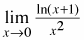3.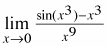4.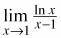5.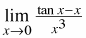6.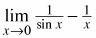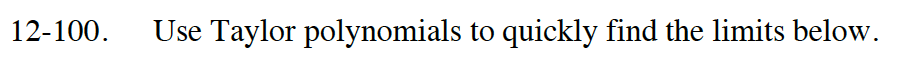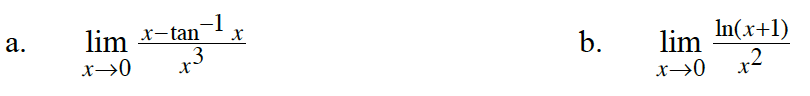$\tan^{-1}(x)=x-\frac{x^3}{3}+\frac{x^5}{5}-...$

$\lim_{x\to 0}\frac{x-\Big(x-\frac{x^3}{3}+\frac{x^5}{5}-...\Big)}{x^3}$

$=\lim_{x\to 0}\frac{x-\frac{x^2}{2}+\frac{x^3}{3}-...}{x^2}$

Use l'Hôpital's Rule once.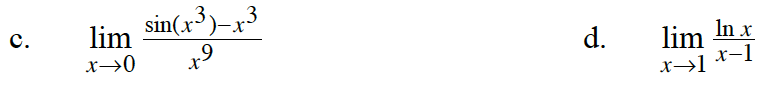$\lim_{x\to 0}\frac{x^3-\frac{(x^3)^3}{3!}+\frac{(x^3)^5}{5!}-...-x^3}{x^9}$

Several iterations of l'Hôpital's Rule can be used. Do you notice a pattern?

This limit is for x → 1, so write the polynomial centered at x = 1.

$\lim_{x\to 1}\frac{(x-1)-\frac{(x-1)^2}{2}+\frac{(x-1)^3}{3}-...}{x-1}$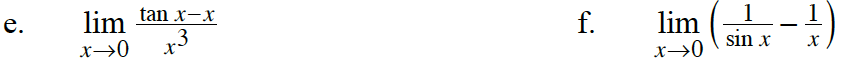$\lim_{x\to 0}\frac{x+\frac{x^3}{3}+\frac{2x^5}{15}+...-x}{x^3}$

$\lim_{x\to 0}\Bigg(\frac{1}{x-\frac{x^3}{3!}+\frac{x^5}{5!}-...}-\frac{1}{x}\Bigg)$

Next, rewrite the argument as a single complex fraction.